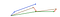# Day 55(DL) — Optimizers for Deep Learning

Earlier we’ve discussed one of the optimization techniques called stochastic gradient descent with momentum. Let’s unravel some more.

Nesterov accelerated gradient(NAG): The intuition behind this approach is “Look before you leap”. In the SGD with momentum, we took two steps(one from the current gradient and the other from past weights) to update the learnable params. One of the shortcomings of this process is there will be many oscillations near the minima points. The reason being, sometimes when we take two steps, the model may overshoot the minima point and again have to come back to the desired region.

• To overcome the limitation of momentum, NAG is introduced. Instead of taking two steps at the same time, we take one step at a time. First, we take a leap based on current_weights — (beta * past_history). This is followed by taking the gradient to do the correction. This method helps the NNs to converge faster by reducing the number of oscillations near the global minima.
• This technique involves having two forward propagations(leap + actual) for one backward propagation.Fig1 — compares SGD with NAG momentum from the original paper
• In the above picture, the blue arrow refers to SGD with momentum. First, the current gradient(small blue vector) is computed followed by the acceleration gained from the previous batches(big blue vector).
• Conversely, the NAG takes a leap based on the previously gained momentum(brown vector), then adjusts itself(green). This would result in a faster convergence rate.

Rprop(Resilient propagation): The idea here is to use only the sign of the gradient rather than the magnitude. The magnitude can vary for different weights and keep changing throughout the learning. This makes it strenuous to pick a single global learning rate.

• Rprop combines the idea of using the sign of gradient along with adapting step size. Increase the step size for a weight multiplicatively (e.g 1.2 times) if the signs of its last two gradients agree. Otherwise, decrease the step size multiplicatively(e.g 0.5 times).
• When the signs are the same(i.e optimum is not crossed yet), the current heading direction is safe and the magnitude of the step size should be increased to reach the optimum point swiftly.
• On the other hand, if the signs are in opposite direction, we have already crossed over the minima. In such scenarios, the step size should be decreased to get back on track.
• The main advantages of this technique are its ability to handle varying gradients across the input predictors and prevent vanishing/exploding gradients.
• But it is not a perfect choice for smaller mini-batches as there will so much noise in the direction of the gradients of each batch.

Adagrad: The intuition behind the approach is every feature has a different weight update. Using the same learning rate across all the predictors will not produce effective learning outcomes. For instance, if an input attribute is sparse, then we can give a high learning rate so that it takes huge steps. While a dense attribute should be given a smaller learning rate in order to make tiny steps ensuring the convergence of minima. The idea here is to adapt the step size based on the individual attribute.

where epsilon is a small value to prevent dividing by zero.

Since we are dividing the term by update history, if there is a frequent change in the value learning rate becomes small(as the denominator will be high). Whereas, if the predictor is updated infrequently, the learning rate will be huge because of the larger steps(momentum).

One of the demerits of this approach is the vanishing gradient. This is due to the fact that the learning rate is vigorously decayed for features with frequent updates. As a result, the gradient steps become meagre thus stopping the learning process completely.

Rmsprop: The problem with the Adagrad is overcome by using Rmsprop where we take an exponentially weighted average of the update history across the batches. This gives control over learning rate decay and thus the aggressive reduction in the learning rate is eliminated. One interesting information about the algorithm is it is not published officially but has been introduced in one of the Coursera courses taken by Geoff Hinton.

Similar to the stochastic gradient with momentum, we give weightage to the past change and the current derivative. The term beta represents how much historical data influences the present details.

Adam(Adaptive Moment Estimation): Finally, Adam combines stochastic gradient momentum with RMS prop for effective results. Even though in RMS prop we change the learning rate in an adaptive manner, including the SGD momentum can further fasten the learning process.

The epsilon takes a small value such as 10^-8, beta1 = 0.9 and beta2 = 0.999. Over many experiments, Adam stands out from the rest of the methods but still based on the problem statement and the nature of the data, the optimum technique can be chosen.